# Decibels: Use with caution

Decibels are commonly used in electronic systems that include RF, audio, and more recently, signal integrity. While many engineers already think in decibels, if you mostly work in the time domain, you may not think the same way. Because much of my background is in RF, I tend to associate the decibel with RF measurements, but the decibel began at audio frequencies defined by telephone system engineers. In the early 1900s, the Bell System had the notion of a mile of standard cable, which corresponded to the loss characteristics of one mile of No. 19 telephone cable [Ref 1]. This concept morphed into the Transmission Unit, defined as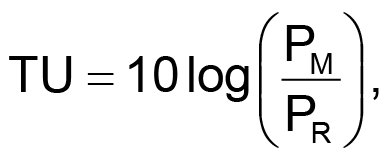where PM is the measured power and PR is the reference power. Later, the TU was given the name decibel, which means a tenth of a bel (named for Alexander Graham Bell) [Ref 2]. Note that they backed into the definition of the bel, which is just the log of the ratio of the two powers. The unit of interest was really the transmission unit and then the decibel. It is tempting to think of the decibel as a unit of measure such as volts or amperes, but it’s a method for handling the ratio of two numbers using base ten logarithms. I noticed that early on, the decibel was abbreviated “db” but more recent usage is “dB.”

If you are interested in drilling deeper into the history of the decibel, the American Radio History web site [Ref 3] has archived many classic technical publications including the Bell System Technical Journal from 1922 and beyond. I spent some time reading through these early articles on telephony and was amazed and humbled by how well electrical principles were understood in those early days [Ref 4].

## Power and voltage

Strictly speaking, decibels are defined in term of power ratios: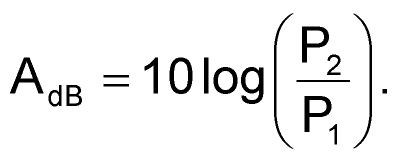Most engineers quickly learn a few rules of thumb to help them with decibel calculations, such as:

• No change in power corresponds to 0 dB
• A factor of 2 in power corresponds to +3 dB or –3 dB
• A factor of 10 in power corresponds to +10 dB or –10 dB

(See Ref. 5 for additional tips on making decibels intuitive.) While decibels are defined as a power ratio, it is common to convert this formula to handle voltage. For RMS voltage,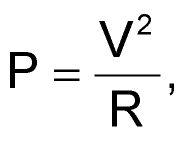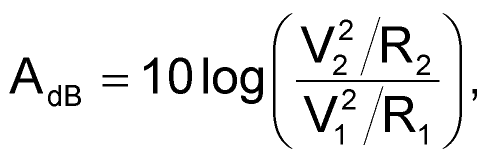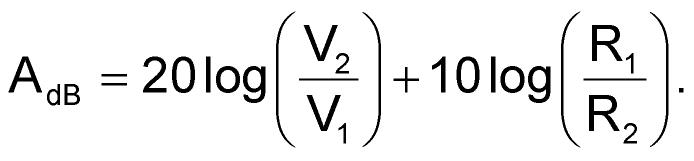A similar formula can be derived for current: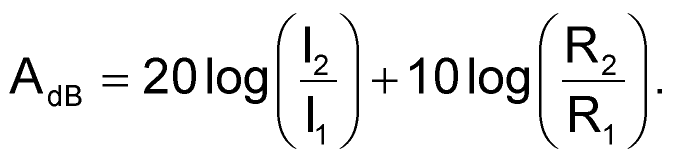In constant impedance (resistance) systems, the ratio R1/R2 equals one and drops out of the equation, leaving the familiar decibel equation for voltage: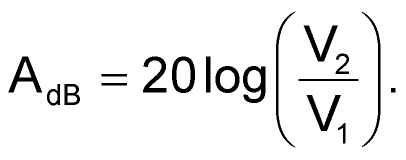Because so many of the signals in electrical engineering are measured in voltage, it is handy to be able to apply decibels to voltage values, instead of power.

• No change in voltage corresponds to 0 dB
• A factor of 2 in voltage corresponds to +6 dB or –6 dB
• A factor of 10 in voltage corresponds to +20 dB or –20 dB

## Use with caution

Ignoring that R1/R2 term can lead to serious confusion, so caution is in order. As an example, let’s consider Figure 1, which shows an amplifier with a high input impedance (R1, 100 kΩ) and a low output impedance driving a load impedance of 100 Ω (R2). The input voltage is 0.1 VRMS and the output voltage is 1 VRMS, for a voltage gain of 10. In decibels, the voltage gain is 20log(10) = 20 dB.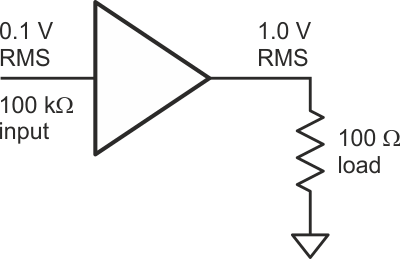Figure 1. An amplifier with 100 kΩ input impedance drives a 100 Ω load.

Now let’s look at the power situation. The input power is (0.1)2/100 k = 0.1 µW, while the power delivered to the load is (1.0)2/100 = 10 mW. Using the power values to calculate the gain, we get 10log(10 mW/0.1 µW) = 50 dB. That causes a different amplifier gain in dB depending on whether we use voltage or power. The decibel formula for voltage ratios was derived from the power formula, so we would expect them to be consistent.

Because we ignored the differences in impedance, we effectively assumed that the impedances are always the same. That’s not true in this case. Going back to the R1/R2 term in the voltage equation, we can calculate the missing factor for the impedance change in Fig. 1. That is,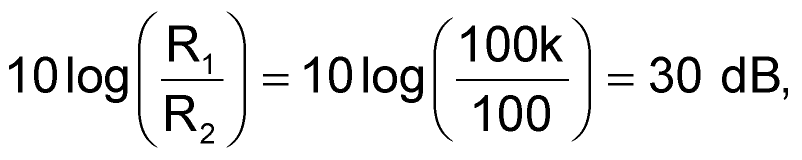which explains the difference between the two gain numbers.

Many systems have consistent impedances. For example, many RF systems are built consistently around a 50 Ω system impedance, with all input, output and transmission line impedances the same. In such a scenario, the power and voltage decibel equations can be used interchangeably, and the decibel numbers track.

In other systems, the impedances vary. You can compensate for this with the R1/R2 correction factor, but more commonly engineers just choose to work exclusively with either voltage or power to avoid any problems. Now, a purist could argue that decibels are defined strictly in terms of power (that’s true) and that all decibel calculations must be done with that in mind. I might agree except that I’ve seen engineers work strictly in terms of voltage and 20log(V2/V1), without a problem. They get the benefits of working in decibels without having to adjust for impedance, but they have to be careful not to mix in power calculations. It is a good example of how engineers use mathematically-impure methods that have practical value.

EDN